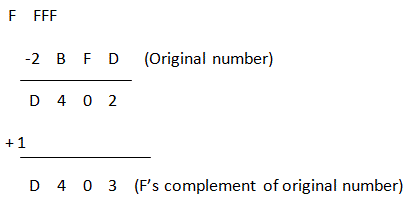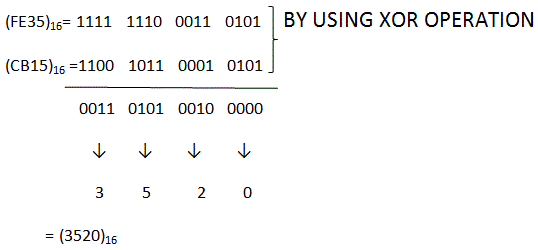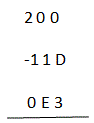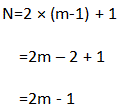# MCQs on Digital Electronics

##### Page 31 of 35. Go to page 1 2 3 4 5 6 7 8 9 10 11 12 13 14 15 16 17 18 19 20 21 22 23 24 25 26 27 28 29 30 31 32 33 34 35
01․ The hex address of the 256th memory location is
00FFF
000FF
00100
001FF

000FF→15×161+15×160=255 As address starts from 000000 location, so 256th memory location will be 000FF.

02․ What are the values of R1 and R2 respectively in the expression (235)R1 = (565)10 = (1065)R2
8, 16
16, 8
6, 16
12, 8

(235) R1 = 2R12 + 3R1 + 5 = 565 …………(I) Solving (I) we will get R1= 16 & -17.5 So by going through the options we will get option (B)

03․ If two numbers in excess-3 code are added and the result is less than 9, then to get equivalent binary
0011 is subtracted
0110 is subtracted

Excess-3 codes are derived from BCD code adding 3 with it. So if we want to get back the equivalent binary from an excess-3 code which is less than 9 we have to subtract 3(0011) from the code.

04․ F’s complement of (2BFD)Hex is
E304
D403
D402
C403

To get F’s complement of any number follow two steps. At first subtract the number from FFFF and then add 1 to the result.05․ The number of digit 1 present in the binary representation of (3 × 512 + 7 × 64 + 5 × 8 + 3) is
6
7
8
9

(3 × 512 + 7 × 64 + 5 × 8 + 3) = 1536 + 448 + 40 + 3 = 2027 (2027)10=(011111101011)2 So we can see from above result the number of digit 1 in binary representation of the given expression is 9.

06․ The minimum decimal equivalent of the number 11C is
183
194
268
269

First check the base of given number and then find the maximum number in that number. In the given number C(13) is the highest number so base ≥ 13. So the minimum number will be (1 × 132) + (1 × 131) + (C × 130) = 169 + 13 + 12 = 194

07․ (FE35)16 XOR (CB15)16 is equal to
(3320)16
(FF35)16
(FF50)16
(3520)1608․ In signed magnitude representation, the binary equivalent of 22.5625 is (the bit before comma represents the sign)
0,10110.1011
0,10110.1001
1,10101.1001
1,10110.1001

(22)10 = (10110)2 (.5625)10 = (1001)2 As 22.5625 is a positive number so its sign bit will contain 0. Thus, binary equivalent of 22.5625 is 0, 10110.1001

09․ Which of the following represents (E3)16 ?
(1CE)16+(A2)16
(1BC)16-(DE)16
(2BC)16-(1DE)16
(200)16-(11D)16

By checking the options we will get,Here subtraction is done in base of 16.So borrow is taken as 16 just like borrow 10 in decimal system) Hence, (200)16 - (11D)16 = (E3)16

10․ To add two m-bit numbers, the required number of half adder is
2m-1
2m-1
2m+1
2m

We know to add two bits we require a half adder and to add three bits we require one full adder or two half adder. To add LSBs of given two m-bit numbers we require only one half adder(as no carry is there) and to add other bits along with carry (generated from previous bit operation) we require one full adder or two half adder. So, total numbers of half adders required adding two m-bit numbers are<<<2930313233>>>